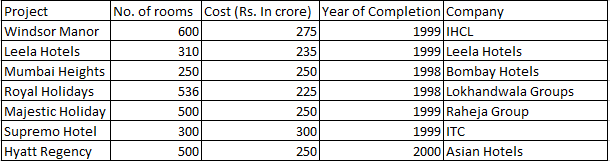### CAT 1997 Question Paper Question 1

Instructions

The following table gives the number of rooms in different hotels in Mumbai and the cost of building each one of them. Note that all the projects started in 1997Note: All projects start in 1997.

Question 1

# Which of the following had the least cost per room?

Solution

The cost per room equals (total cost)/(total rooms)
To calculate the least cost per room, we have to only check IHCL and Lokhandwala Group. A simple inspection will remove all other companies as they have more cost and lesser rooms than either of the two.

Cost per room of IHCL = 275/600 = 45.83 lakhs
Cost per room of Lokhandwala = 225/536 = 41.97 lakhs.

Hence, the cost per room is least for Lokhandwala Group.